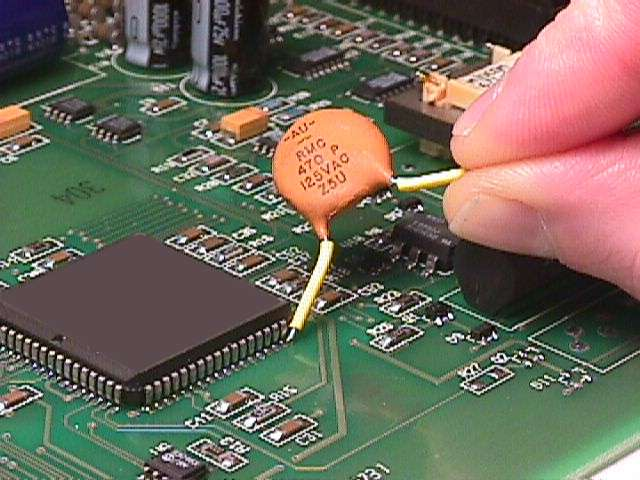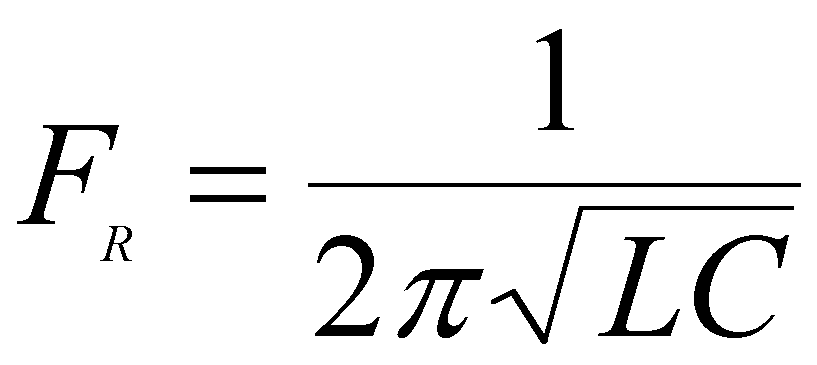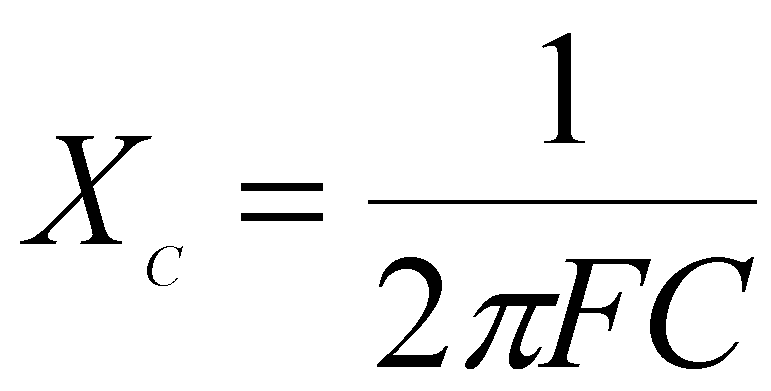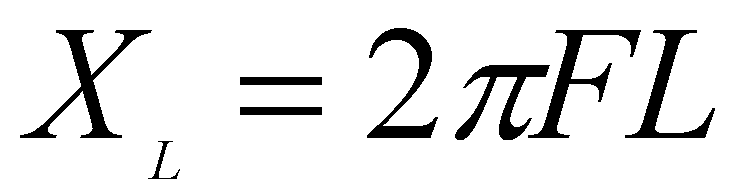High Frequency Measurements Web Page
Douglas C. Smith

Address:  P. O. Box 1457, Los Gatos, CA 95031
TEL:      800-323-3956/408-356-4186
FAX:      408-358-3799
Mobile:   408-858-4528
URL:      www.dsmith.org
Email:    doug@dsmith.org

Technical Tidbit - April 2001
Measurement Error Caused by Probe Input ImpedanceFigure 1. Not a good way to probe a high speed signal!

You wouldn't measure a high speed logic signal using the technique shown in Figure 1, of course. But, sometimes scope probes, even high performance FET ones, can have a similar, significant loading effect on the circuit. All measurements must involve a loop comprised of the probe tip, ground connection (other probe tip connection for balanced measurements), and some path length to the source on the circuit being measured. It is not difficult for this measurement loop to have 20 nH for many FET probe configurations. The University of Missouri at Rolla has a good inductance calculator. To access this tool, click here. For round numbers, let's assume a probe input capacitance of 1 pF although some FET probes can be lower. I have seen some FET probes recently in the 0.6 pF range.

Equation (1) below shows the resonant frequency of a tuned circuit. If we put in 20 nH and 1 pF the result is only about 1.125 GHz.(1)

The capacitive reactance of the probe's input capacitance is given by equation (2). At 1.125 GHz, for 1 pF, Xc is only about 140 Ohms and represents essentially the input impedance of the probe. This input impedance is not much higher than many of the signals likely to be probed on a printed wiring board. It generally does not meet the criteria that the probe be ten times the circuit impedance so as not to unduly affect the circuit.(2)

Compared to the relatively high input resistance of the probe (usually 10k Ohms or higher) this reactance forms a fairly high Q tuned circuit that can give overshoot and ringing to an observed waveform edge.

The inductive reactance of 20 nH is given by equation (3) and is also about 140 Ohms at 1.125 GHz. When added to the input impedance of the probe (140 Ohms of capacitive reactance), the loading on the circuit in this example is near zero Ohms (just the series resistance in the circuit) at 1.125 GHz!(3)

If we assume circuit impedance on the order of 50 Ohms for a printed wiring board and would like the probe to be ten times the circuit impedance, then the probe should have a 500 Ohm input impedance at the highest frequency at which the signal has significant components. For a 1 pF probe, that frequency would be about 318 MHz from equation (2). Even at 0.6 pF for the probe input capacitance, the frequency meeting the criteria is only about 530 MHz! One can argue that ten times the circuit impedance may not always be needed. But, even just requiring only four times circuit impedance means that our hypothetical 0.6 pF probe is useful up to only about 1.3 GHz!

Bandwidth specifications on oscilloscope probes can often sound impressive, but it is important to take into account circuit loading when selecting a probe to measure high frequency signals. Input impedance information is usually available for most probes. It is as important as the bandwidth specification.

Questions or suggestions? Contact me at doug@dsmith.org